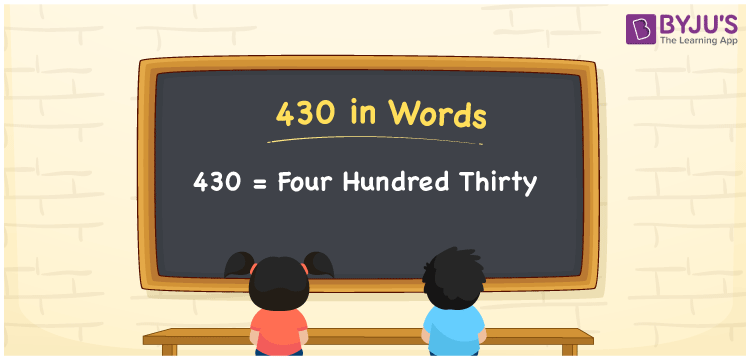# 430 in Words

430 in words can be written as Four Hundred Thirty. The concepts like counting numbers which are important from primary education are discussed here in brief. For instance, if you spend Rs. 430 in buying a 1 kg of fish, then you can say that “I bought a 1 kg of fish for Four Hundred Thirty Rupees”. The English alphabet is used to write 430 in words. Students can get a clear knowledge of numbers in words with the help of the study materials given at BYJU’S. The number 430 can be read as “Four Hundred Thirty” in English.

 430 in words Four Hundred Thirty Four Hundred Thirtyin Numbers 430

## 430 in English Words## How to Write 430 in Words?

Place value chart and expanded form of 430 can be learnt easily in this section. There are three digits in the number 430. The place value chart for 430 is provided below for reference to help students understand the concepts effectively.

 Hundreds Tens Ones 4 3 0

The expanded form of 430 is provided below:

4 × Hundred + 3 × Ten + 0 × One

= 4 × 100 + 3 × 10 + 0 × 1

= 430

= Three Hundred Twenty

Hence, 430 in words is written as Three Hundred Twenty.

430 is a natural number that precedes 431 and succeeds 429.

430 in words – Four Hundred Thirty

Is 430 an odd number? – No

Is 430 an even number? – Yes

Is 430 a perfect square number? – No

Is 430 a perfect cube number? – No

Is 430 a prime number? – No

Is 430 a composite number? – Yes

## Frequently Asked Questions on 430 in Words

Q1

### How can 430 be written in words?

430 can be written as “Four Hundred Thirty” in words.
Q2

### Is 430 an odd number?

No, 430 is not an odd number because it is divisible by 2.
430/2 = 215
Q3

### Write Four Hundred Thirty in numbers.

Four Hundred Thirty can be written in numbers as 430.